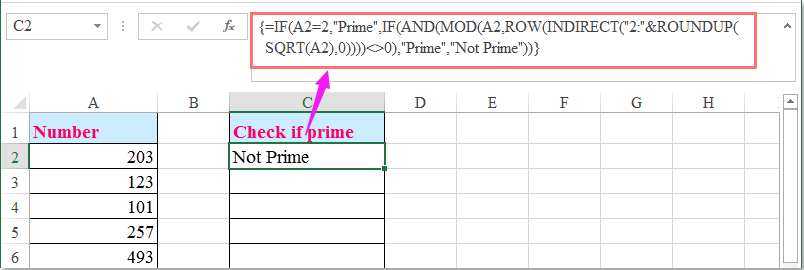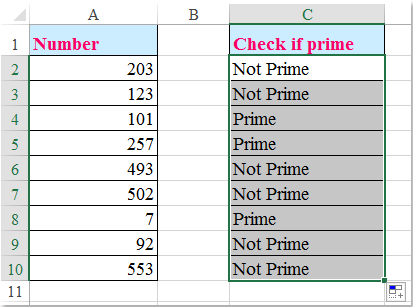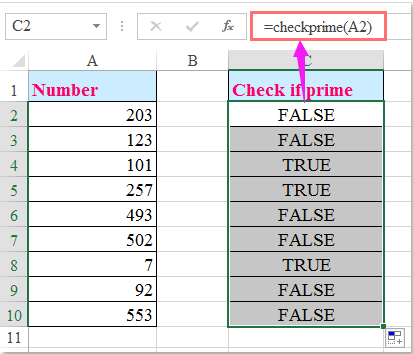oror

## 如何檢查數字是Excel中的素數？

####檢查數字是否是數組公式的素數###### 節省50％的時間，每天為您減少數千次鼠標點擊！

1。 在空白單元格中輸入以下公式 - 例如在數據旁邊的C2：

=IF(A2=2,"Prime",IF(AND(MOD(A2,ROW(INDIRECT("2:"&ROUNDUP(SQRT(A2),0))))<>0),"Prime","Not Prime")) (A2 是單元格包含您想要檢查的號碼），然後按 Ctrl + Shift + Enter 如果數字為素數，則會在單元格中顯示“Prime”，否則將顯示“Not Prime”，請參閱屏幕截圖：2。 然後選擇單元格C2，然後將填充手柄向下拖動到您要應用此公式的單元格，如果是素數，則所有數字都將被識別。 看截圖：####用用戶定義函數檢查一個數字是否是質數

1。 按住 ALT + F11 鍵打開 Microsoft Visual Basic for Applications 窗口。

2。 點擊 插入 > 模塊，並將以下代碼粘貼到 模塊窗口.

VBA代碼：檢查數字是否為素數：

```Function CheckPrime(Numb As Single) As Boolean
'Updateby Extendoffice 20160614
Dim X As Long
If Numb < 2 Or (Numb <> 2 And Numb Mod 2 = 0) _
Or Numb <> Int(Numb) Then Exit Function
For X = 3 To Sqr(Numb) Step 2
If Numb Mod X = 0 Then Exit Function
Next
CheckPrime = True
End Function
```

3。 然後保存並關閉此代碼，返回到工作表，並輸入此公式： = checkprime（A2） 進入數字列表旁邊的空白單元格，然後將填充手柄向下拖到要包含此公式的單元格中，如果數字是質數，則它將顯示TRUE，否則將顯示FALSE，請參見截圖：### Kutools for Excel解決了您的大多數問題，並使您的生產率提高了80％

• 重用: 快速插入 複雜的公式，圖表 以及你以前用過的任何東西; 加密單元格 密碼; 創建郵件列表 並發送電子郵件...
• 超級方程式酒吧 （輕鬆編輯多行文字和公式）; 閱讀佈局 （輕鬆讀取和編輯大量單元格）; 粘貼到過濾範圍...
• 合併單元格/行/列 不丟失數據; 分裂細胞含量; 組合重複的行/列...防止重複的細胞; 比較範圍...
• 選擇複製或唯一 行; 選擇空行 （所有細胞都是空的）; 超級查找和模糊查找 在許多工作簿中; 隨機選擇......
• 精確複製 多個單元格而不更改公式參考; 自動創建參考 多張表; 插入項目符號，複選框等等......
• 提取文本，添加文本，按位置刪除， 刪除空間; 創建和打印分頁小計; 在單元格內容和註釋之間轉換...
• 超級過濾器 （將過濾方案保存並應用到其他工作表）; 高級排序 按月/週/日，頻率等; 特殊過濾器 用粗體，斜體......
• 結合工作簿和工作表; 根據鍵列合併表; 將數據拆分為多個表格; 批量轉換xls，xlsx和PDF...
• 超過300強大的功能。 支持Office / Excel 2007-2019和365。 支持所有語言。 在您的企業或組織中輕鬆部署。 全功能30天免費試用。### Office選項卡為Office提供選項卡式界面，使您的工作更輕鬆

• 在Word，Excel，PowerPoint中啟用選項卡式編輯和閱讀，Publisher，Access，Visio和Project。
• 在同一窗口的新選項卡中打開並創建多個文檔，而不是在新窗口中。
• 通過50％提高您的工作效率，每天為您減少數百次鼠標點擊！Say something here...
symbols left.
###### or post as a guest, but your post won't be published automatically.
• To post as a guest, your comment is unpublished.
· 4 months ago
Yes it worked wen I did Ctrl+Shift+Enter... !! Thank you..:D and Do u mind explaining the algorithm please.
• To post as a guest, your comment is unpublished.
· 6 months ago
There's a reference error when you type in numbers that have more than 12 digits.
• To post as a guest, your comment is unpublished.
· 6 months ago
Hello,Nathan,
As you said, when the numbers are longer than 12 digits, it will become scientific notation. The formula is not applied for this formatting.
• To post as a guest, your comment is unpublished.
· 11 months ago
well 4095 is not a prime. still it gives result as prime no though. its incorrect.
• To post as a guest, your comment is unpublished.
· 11 months ago
Hello, vaibhav,
When you pasting above formula, you should press Ctrl + Shift + Enter keys together, not just Enter key, please try it again.
• To post as a guest, your comment is unpublished.
· 2 years ago
Your formula is not working. Eg. 1681 is NOT a prime but by formula it is.
=IF(A2=2,"Prime",IF(AND(MOD(A2,ROW(INDIRECT("2:"&ROUNDUP(SQRT(A2),0))))<>0),"Prime","Not Prime"))
• To post as a guest, your comment is unpublished.
· 2 years ago
Hello, Jan,
The above formula is correct, after pastingt the formula into a cell, you should press Ctrl + Shift + Enter keys together, not just Enter key.
Please try it again, thank you!
• To post as a guest, your comment is unpublished.
· 2 years ago
[b]this is my fastest version[/b]

starting_number = 1 'input value here
last_number = 30000 'input value here
For a = starting_number To last_number
c = a
For b = 2 To c
If a Mod b = 0 And c b Then
Exit For
Else
If b = c Then
Exit For
Else
If Round(a / (b + 1)) + 1 > b Then
c = Round(a / (b + 1)) + 1
End If
End If
End If
Next b
Next a
End Sub

Sub ISPRIME3()
number_to_be_checked = 2000000000 'input value here
c = number_to_be_checked
For b = 2 To c
If number_to_be_checked Mod b = 0 And c b Then
MsgBox "Not Prime. Divisible by " & b
Exit Sub
Else
If b = c Then
MsgBox "Prime"
Exit Sub
Else
If Round(number_to_be_checked / (b + 1)) + 1 > b Then
c = Round(number_to_be_checked / (b + 1)) + 1
End If
End If
End If
Next b
End Sub
• To post as a guest, your comment is unpublished.
· 2 years ago
The user defined function does not seem to work for numbers above ~16777213
• To post as a guest, your comment is unpublished.
· 2 years ago
starting_number = 99990 'input value here
last_number = 99999 'input value here
For a = starting_number To last_number
For b = 2 To a
If a - b * Int(a / b) = 0 And a b Then
Exit For
Else
If a = b Then
End If
End If
Next b
Next a
End Sub

For a = starting_number To last_number
For b = 2 To a
If a - b * Int(a / b) = 0 And a b Then
Exit For
Else
If a = b Then
End If
End If
Next b
Next a
End Function

'to check if a number is prime
Sub ISPRIME()
number_to_be_checked = 102 'input value here
For b = 2 To number_to_be_checked
If number_to_be_checked - b * Int(number_to_be_checked / b) = 0 And _
number_to_be_checked b Then
MsgBox "Not Prime. Divisible by " & b
Exit Sub
Else
If number_to_be_checked = b Then
MsgBox "Prime"
End If
End If
Next b
End Sub

'to check if a number is prime
Function ISPRIME2(number_to_be_checked)
For b = 2 To number_to_be_checked
If number_to_be_checked - b * Int(number_to_be_checked / b) = 0 And _
number_to_be_checked b Then
ISPRIME2 = "Not Prime. Divisible by " & b
Exit Function
Else
If number_to_be_checked = b Then
ISPRIME2 = "Prime"
End If
End If
Next b
End Function
• To post as a guest, your comment is unpublished.
· 2 years ago
The array formula doesn't work but the User defined function does and was very helpful. Thanks!
• To post as a guest, your comment is unpublished.
· 2 years ago
The array formula above doesn't work but the User Defined function does and was very helpful.Thanks!
• To post as a guest, your comment is unpublished.
· 2 years ago
Hi there,
I like your article. Thanks a lot.
My simple tests showed that 99 was a prime number while 99 is not as 99 is divided by 3 and 33 as well as 1 and 99.
Can you let me know what is wrong with my operation?